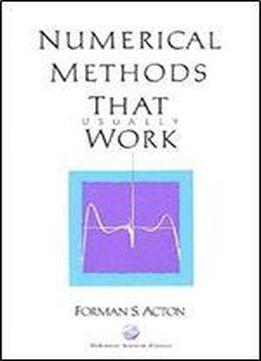# Numerical Methods That Work by Forman S. Acton / 1997 / English / PDF

Numerical Methods that Work, originally published in 1970, has been reissued by the MAA with a new preface and some additional problems. Acton deals with a commonsense approach to numerical algorithms for the solution of equations: algebraic, transcendental, and differential. He assumes that a computer is available for performing the bulk of the arithmetic. The book is divided into two parts, either of which could form the basis of a one-semester course in numerical methods. Part I discusses most of the standard techniques: roots of transcendental equations, roots of polynomials, eigenvalues of symmetric matrices, and so on. Part II cuts across the basic tools, stressing such commonplace problems as extrapolation, removal of singularities, and loss of significant figures. The book is written with clarity and precision, intended for practical rather than theoretical use. This book will interest mathematicians, both pure and applied, as well as any scientist or engineer working with numerical problems.

views: 432